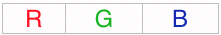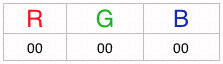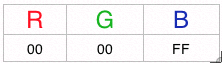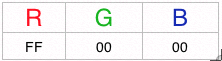# Hex Colour Codes and Colour

As we all know that hexadecimal codes are used to create colours that we see on the monitor and are used widely be web developers all over the world. Now we know how to create numbers with hex codes, but here's how you can create colour codes (well basic colour codes anyways).

Monitors use RGB format, which stands for red, green and blue. There are the primary colours that are used to make light, when these lights come together they create the colour that is supposed to get created. So the hex colour codes tell how these lights need to behave (in html and photo editing). So let's take this step by step:

1. Imagine there are three columns with Red Green And Blue.2. In each column there are two digits, you cannot have one digit only two digits. Why? Because there are only 255 combinations of each colour (255 is the same as 16x15 + 1x15) which means that you can only have 2 spaces, which put together give millions of combinations.
For example:3. Since f=15 and, if any value is f that is f should be on. For example: If I want the colour to be blue, I will have f under the blue column, and since you can put 2 digits you can put 2 f's in there so that the pixel becomes completely blue!4. If you want any pixel to be turned off, the numbers that you put under the column should be 00 (0's have no value, which tells the pixel that it should not use that colour)For example: The picture shows that only red is being used (because it has the highest value of 255, and the others aren't being used because it is a 0.
5. When the quantities of these colurs are mixed together you get different light (for example if all of them are on, the colour we see would be white, and if all of them are off the colour we see would be black)
Hex colour codes are basically all the numbers under all of the three colums above (RGB) put together. So the hex code for green would be : 00ff00 (look at the picture below to help you understand)

Then all you do is use these colour codes using the following tags "(font color=######) and type in youre text inbetween and then when you don't want the colour any more just end it with (/font). Note: You NEED to replace the '(' brackets with '<' brackets for it work. And put in the hex code where it says '######'!

These days nobody uses their brain to think of hex color codes because they are done for you by a lot of programs (such as Adobe Photoshop) that you can find easily. However I still think it is interesting to know how cool the computer codes are, so it's don't consider this post a waste of your time... any gaining knowledge is not wasting time!

Got a question, tip or comment? Send them to beyondteck+question@gmail.com and we'll try to answer it in a blog post!